## 6.11Turbulent mixing

Turbulent ﬂow is characterised by signiﬁcant mixing of ﬂuid eddies as the Reynolds experiment in Sec. 6.1 shows. CFD simulations generally need to accommodate turbulent mixing since it inﬂuences the diﬀusion of mass, momentum and energy. While the ﬂuid mixing by mass diﬀusion itself can be important, the eﬀect on momentum diﬀusion is often critical because it impacts the calculation of viscous forces and, thus, the ﬂow itself.

### Eddy viscosity

Boussinesq was the ﬁrst to devise a model for turbulence. He recognised the similarity between the random motion of both eddies in a turbulent ﬂuid and particles at a molecular scale.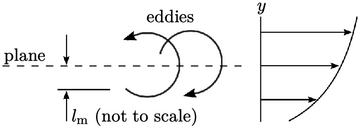By analogy to kinetic theory in Sec. 6.10 , shear stresses due to turbulence are caused by the net momentum, tangential to a plane, due to the motion of eddies. Boussinesq related this shear stress to the velocity gradient through an eddy viscosity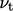.14

He presented the turbulent stress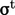in tensor form, including a pressure. Kinetic theory relates pressure to ﬂuctuations15 in particle velocity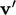by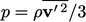; the kinetic energy associated with the ﬂuctuations is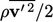. Applying the same argument to velocity ﬂuctuations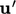due to turbulence, leads to a turbulent “pressure”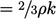, where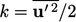is the turbulent kinetic energy per unit mass.

By analogy with the Newtonian ﬂuid model Eq. (2.41 ), the eddy viscosity model of Boussinesq, incorporating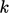, is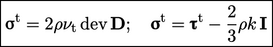(6.20)
where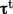is the viscous component of Reynolds stress and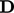is the deformation rate tensor deﬁned in Eq. (2.33 ). Inevitably Eq. (6.20 ) and Eq. (2.41 ) closely resemble one another.

The model of Eq. (6.20) requires some means of calculating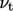. Kinetic theory gives a quantitative prediction of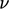in Eq. (6.19 ) which led Boussinesq to hypothesise that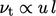, where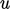andare a representative speed and length, respectively, with the speedrelating to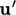due to turbulence.

The turbulent viscosity can also be expressed as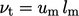by absorbing the constant of proportionality within a characteristic speed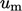and a mixing length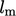, discussed in Sec. 6.12 .

Eddy viscosity and mixing length are useful concepts in turbulence modelling. However, it should be recognised that there are limitations in the analogy with kinetic theory, e.g.:

• momentum is exchanged between submicroscopic particles through intermittent, discrete collisions, compared to the continuous interaction between eddies;
• the magnitude of random particle motions is generally equal in all directions, whereas the level of turbulent ﬂuctuations can sometimes vary signiﬁcantly with direction.

14Joseph Boussinesq, Essai sur la théorie des eaux courantes, 1877.
15Daniel Bernoulli, Hydrodynamica, sive de viribus et motibus ﬂuidorum commentarii, 1738.

Notes on CFD: General Principles - 6.11 Turbulent mixing